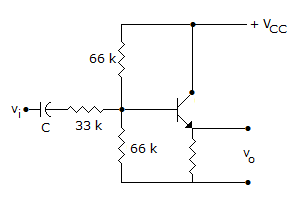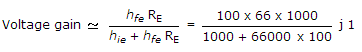# Electronics and Communication Engineering - Analog Electronics

21.

In the amplifier circuit of figure hfe = 100 and hie = 1000 Ω. The voltage gain of amplifier is aboutA. 0.33 B. 0.5 C. 0.66 D. 1

Explanation:.

22.

The efficiency of a full wave rectifier using centre tapped transformer is twice that in full wave bridge rectifier.

 A. True B. False

Explanation:

Efficiency of full wave rectifier with centre tapped transformer is slightly higher than that of bridge rectifier.

23.

Assertion (A): CE amplifier is the most widely used BJT amplifier

Reason (R): CE amplifier has zero phase difference between input and output

 A. Both A and R are correct and R is correct explanation for A B. Both A and R are correct but R is not correct explanation for A C. A is correct R is wrong D. A is wrong R is correct

Explanation:

There is 180° phase difference between input and output.

24.

Assertion (A): For large signal variations an amplifier circuit has to be analysed graphically

Reason (R): The output characteristics of a transistor is nonlinear.

 A. Both A and R are correct and R is correct explanation for A B. Both A and R are correct but R is not correct explanation for A C. A is correct R is wrong D. A is wrong R is correct

Explanation:

Since the characteristics is non-linear, graphical analysis is needed.

25.

Negative feedback reduces noise originating at the amplifier input.

 A. True B. False